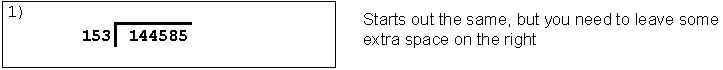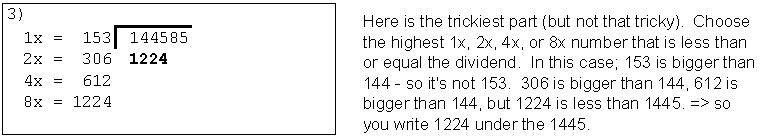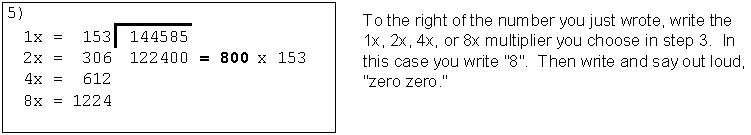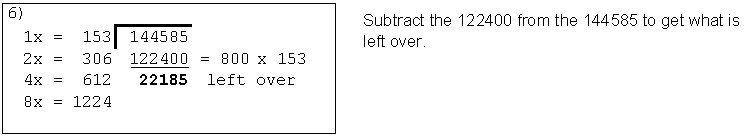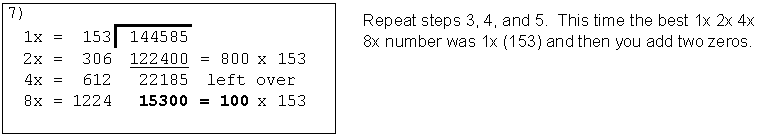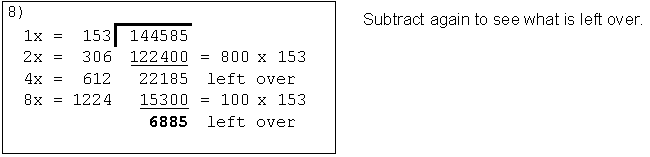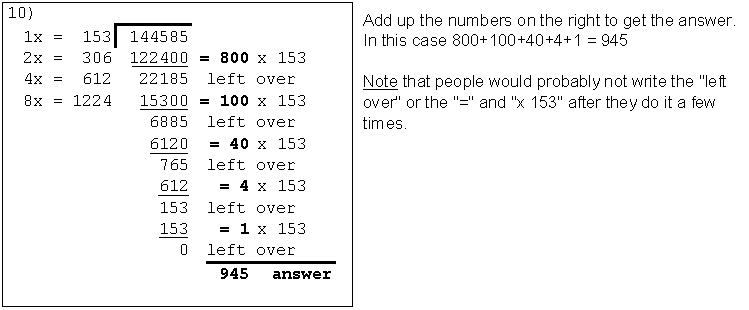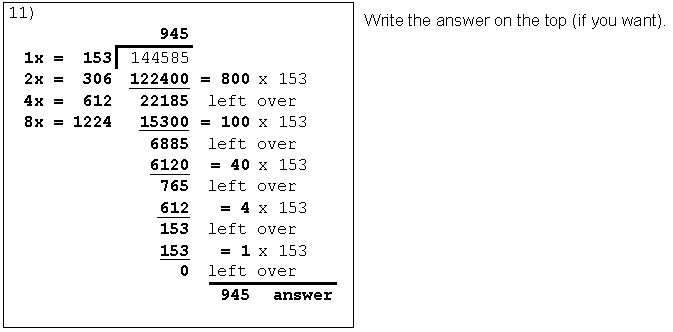# Long Division Teaching Aid, "Double Division"

Alternative name: "1-2-4-8 Division"
Alternative name: "Decimal Double Division"
Jeff Wilson   9/29/2005   ( site visits)

Teaching Double Division can help in teaching long division by reinforcing the principles of division and giving students success with a less frustrating alternative.

Double Division does not depend on memorizing the multiplication facts or estimating how many times one number goes into another. It may take 50% longer, but it is far less frustrating and probably easier to understand than Long Division. example

It's Easy!Step 1 - Double, double, double.
Step 2 - Subtract off multiples.From the class room:(1)  "This is actually fun!"(2)  "More right answers than wrong!"(3)  What about 8.268 / 3.5?

Click "Step 1" on the calculator (above right) for step-by-step instructions.

Double Division may be easier to understand than Long Division because it deals with whole numbers rather than individual digits. You can see multiples of the divisor being subtracted from the dividend. In the example to the right, you see that 37 x 2,000 = 74,000 is being subtracted from the dividend of 85,434. (better notation?). With Long Division you'd be subtracting 74 from 85; neither of which are real numbers in the problem.

Extension to decimals (April 2008):
To extend the answer by one decimal place, start again with the remainder times ten. Repeat this as many times as you'd like, or until a remainder repeats itself. Decimal Examples

DOUBLE DIVISION DISCUSSIONS: (others?)

Video on Double Division at LearnZillion.com.

Writen procedure below,  Bigger calculator here.

Long Division and Double Division side-by-side.

Decimal examples: remainder to decimal  neg powers of 10

Webpage on the long division procedure.

Paper on teaching long division.

This method is a God send. This method sucks!

Funny video on new math.Double Division Help Calculator
Fill in known the values and
click Step 1, Next, Next,,,
 R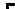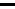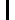Each step is explained here, and
the step is highlighted below.
 Double Division Help Calculator to help show steps of double division. (Long Division Help Calculator showing steps of long division - COMING SOON.)
 WHAT THE BUTTONS DO: - Step 1 => Enter numbers and click this to start the problem. - Next Step => Does next step of the problem with explanation above. - Back => Clicking this backs up one step. - Finish => Click finish and the problem will be completed to the end.
 feedback © Copyright 2006bigger one
View the log file for this website to see from where people are coming from, how long they stay, what buttons they press, and what links they click on.

INTEREST GROWING:MOTIVATION:

The value of teaching manual division is to give people a method they can actually use if they have to, and to teach about mathematics.

It could be argued that if students seldom use long division after leaving school then it might be better for them to have a simpler and more intuitive method at their disposal - one that is easier to remember and understand. Would someone ten years out of school have an easier time doing long division or Double Division? - I'm not sure, but Double Division seems simpler to me.

About teaching math, I'm not sure that long division teaches math very well. It helps teach people how to follow a long procedure and it gives some practice multiplying and subtracting. I'm not sure how many people understand what is happening when you bring down the next digit or understand why you have to add a zero to the answer when the "number after subtracting" is less than the divisor.

One problem I see with Double Division is that you have to do more subtraction. I personally find subtraction harder than multiplication. The other problem is that (not counting the trial and error) Double Division will usually have more steps.

WHAT'S THE DIFFERENCE?

1) In long division you guess which multiple to subtract, where as in Double Division you pick from four options. (Any multiple you pick works. You don't always have to pick the largest possible one; sometimes I pick the easiest one to subtract.)

2) In Double Division you write out the zeros so it's clear how big the numbers are.

3) In Double Division you write your answer on the right side where you have room to accumulate many parts of the answer. In long division you write the answer on the top and have to get each digit of the answer exactly right - because there is only room there to write one number.

Here is how I see it so far:

REASONS TO TEACH DIVISION:

- to teach mathematics

- a method to actually use in rare instances

- to prepare students for higher math

- teaches how division works

- no trial and error: I don't know how many times 372 go into 2711.

- easier to do: Double, double, double, subtract the best ones, add up the answer.

- gives practice doubling numbers

- more subtraction

- more steps (see note below)

- requires more space on the paper

- incremental difficulty in going on to decimals ?may? be more

- may not lead as directly to polynomial division - arguable

IS Double DIVISION LONGER?

If we assume there is an equal chance of all ten digits being in the answer then on average there will be 1.5X as many "multiple and subtract" steps. For example a "7" in the answer requires 3 steps, and a zero in the answer requires no steps.

Also remember that the multiply part of the "multiply and subtract" steps is already done for you. So this part will be faster. Of course you have to pre-multiple the divisor three times in the beginning.

In the end I think it is longer, but not as much as you might think initially.

FEEDBACK SO FAR - 10/14/2005

The response has been mixed. A few people have thought doubling the divisor three times was an efficient way to get trial multiples, and liked the idea of not having to guess. Several people have said this method is no easier than long division. I think the challenge is comparing the "easiness" of one thing that you know to another thing you are just learning. But on the other hand, this explanation of long division is pretty simple.

On October 11, 2005 a sixth grade teacher posted this comment after teaching Double Division to his class.

STEP-BY-STEP